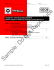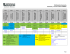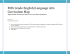# Document 6518289

## Transcription

Document 6518289
```Teacher
2-3 weeks of instruction (2nd-4th
weeks of March 2015)
CCSS.Math.Content.7.G.A.1 Solve problems involving scale drawings of geometric figures, including computing actual
lengths and areas from a scale drawing and reproducing a scale drawing at a different scale.
Mrs. Chasity Shipley
Demonstrators/ Exit
Standards
(Core Content)
Subject
Geometry 2D
Timeline (Dates)

CCSS.Math.Content.7.G.A.2 Draw (freehand, with ruler and protractor, and with technology) geometric shapes with given
conditions. Focus on constructing triangles from three measures of angles or sides, noticing when the conditions determine
a unique triangle, more than one triangle, or no triangle.

CCSS.Math.Content.7.G.B.5 Use facts about supplementary, complementary, vertical, and adjacent angles in a multi-step
problem to write and solve simple equations for an unknown angle in a figure.
Essential
Question(s)
What is the difference between 2D and 3D figures? How can I measure and create 2D figures to solve real world
problems?
Guiding Questions:
(Objectives,
Learning Targets)
1. I can classify angles as acute, right, obtuse, or straight.
2. I can measure and create angles using a protractor.
3. I can determine unknown angle measures by writing and solving
simple equations. This is based on knowledge of supplementary,
complementary, vertical, adjacent, and corresponding angles.
4. I can classify and draw (with appropriate tools such as rulers,
protractors, technology, etc) polygons using given conditions.
5. I can construct triangles from given angle or side measures and
determine if it is a unique triangle, more than one triangle, or no
triangle at all.
6. I can use correct notation to name geometric elements and make
statements of similarity and congruency.
7. I can solve proportional problems that involve scale drawings of
geometric figures by computing actual lengths and areas from a scale
drawing.
Unit
Vocabulary
Angle
Point
Ray
Line segment
Supplementary
Complementary
Vertical
Corresponding
Similar
Congruent
Protractor
Degree
Straight angle
Right angle
Obtuse angle
Acute angle
Scalene
Equilateral
Isosceles
Scale factor
8. I can construct a scale drawing that is proportional to a given
geometric figure using an appropriate scale.
1
What products will students create to show they understand? (Formative & Summative Assessments)
Please attach Open Response and Rubric.
Manipulative Problem-Solving
Quizzes
Pre-Post Assessments
Daily Skills Checks -Warm-ups
Open Response
Hook :
Use a geometric brainteaser. “How man many triangles are in the picture?” Students use embedded triangles to find the total.
Mastery
Thoughtful Education Strategies (Minimum of three per week) (Fifteen different strategies per semester)
Interpersonal
Understanding
Self – Expressive
Utility (Can be used in multiple styles)
Fact or Fiction
Categories
Spider/Fist List
Memory Box
Word Association Write to learn
Word Wall
Building writing
Interactive Lecture
Group & Labeling
Student Activities Below
Day 1: Geometry Vocab
and symbols
- Go to the lab with student
handout (vocabulary notebook)
and allow students to use the
interactive dictionary to
discover the terms.
with the vocab notebook.
there will be a vocab and
symbols test at the end of the
chapter.
www.amathsdictionaryforkids.c
om/dictionary.html
Reciprocal Learning
Think/Pair/Share
Give one, Get one
Collaborative Summarizing
Jeopardy
Anticipation Guides
KWL
Concept Attainment
Compare/Contrast
1,2,3,4
Yes, No, Why
Day 2 Classifying and
Constructing Angles (1-2
days)
- warm-up
- students take notes on types of
angles and use the flipchart for
examples.
- Using the online model, the
teacher models how to
effectively measure angles.
http://www.teacherled.com/reso
urces/anglemeasure/anglemeas
Day 3: Notations
Day 4: Angle
Relationships (2-3 days)
- model notating of the
following: lines, angles, rays,
segments, perpendicular lines,
parallel lines, congruent
triangles, similar triangles,
measurement of angles, etc.
- Students complete a Bridge to
Algebra worksheet.
- Model corresponding,
supplementary, complementary,
Students must use
EQUATIONS to find missing
values.
- Use IXL for practice in
computer lab
- Students measure angles from
the Bridge to Algebra practice
sheet. On the back of the sheet
(blank paper), students are
angle degrees. The teacher
models how to construct angles.
Students switch papers and
measure their classmate’s angle
constructions for accuracy.
*If time is limited, this could be
easily embedded into warm-ups
and other lessons.
Etch-a-Sketch
Mystery
1,2,3,4
Boggle
Comprehension
Jeopardy
Voc Notebook
Carousel Brainstorming
Boggle
Vocabulary Code
Jigsaw
4-2-1 Free Write
Kindling
TGT
Day 5: Polygons(classifying
and angles) (1-2 days)
- Think/Pair/Share- students
list the correct names for 3-10
sided polygons. A big
misconception is that a four
sided figure is called a square.
Discuss regular vs irregular
figures
- Vocab: vertex,vertices, edges,
interior angle, polygon, regular
polygon
2
8-2 textbook
No homework
CORE STANDARDS for above lessons
CCSS.Math.Content.7.G.A.1 CCSS.Math.Content.7.G.A.2
CCSS.Math.Content.7.G.A.2 .
CCSS.Math.Content.7.G.B.5
Student Activities
Day 6 Triangles(Classifying
Day 7: Triangle
and Finding missing degrees)
Inequality/Spaghetti Lab
(1-2 days)
- Students will know if three
- Using a foldable(located in P side lengths forms a triangle.
drive), students will classify
Students will “discover” the
triangles by sides and angles.
pattern. Students will work in
Students will brainstorm
groups of 2. At the end,
impossible triangle types. Also explain that there are no
include 180 degrees within a
“bullies” allowed in triangle
triangle. Model how to find
side lengths. The two smaller
missing angles using equations
sides must be capable of
and angles such as 3x, 2x, 5x to overtaking the big one.
find the missing angles.
- homework: practice
classification and missing
measures
8-3 textbook
textbook 8-5
CCSS.Math.Content.7.G.A.2
CCSS.Math.Content.7.G.B.5
CCSS.Math.Content.7.G.B.
5
CCSS.Math.Content.7.G.A.
2
Day 8: Classifying
Day 9: Constructions (2 days)
Day 10: Similar Figures
(QZ Enrichment)
tree on the Pdrive to explain the
characteristics. Don’t forget to
include Kite. Use kutasoftware
to practice.
Materials: Student handout and
a 3-4 uncooked spaghetti
noodles per student.
Worksheet on P-drive for
practice
Textbook 8-7
Materials: protractor, compass,
pencil, and construction
worksheet
Hook: Present students with a
problem. “On a piece of
notebook paper, construct a
triangle measuring 4 inches, 5
inches, and 7 inches.” Students
will realize the difficulty
without knowing the angleTex
degrees. Transition into using a
compass….
The teacher models how to
construct the first figure.
Students use appropriate tools
to make the first construction
following the teacher’s
demonstration. Repeat this for
all constructions.
-warm-up: discuss congruent
figures and how to notate.
- Using the textbook’s
powerpoint on this lesson,
model how to notate similar
figures and to use proportions
to find missing side lengths.
- workbook practice problems
textbook: 5-7 and 5-8
Day 2: Teacher facilitates
while students complete
constructions without the
teacher demonstrations.
“Constructions 2” can be found
on the pdrive. Peer revision:
3
Students switch papers and
offer feedback before turning
constructions in. Most students
will fail to label vertices, sides,
and angles.
-textbook 8-6
CORE STANDARDS for above lessons
CCSS.Math.Content.7.G.A.2
CCSS.Math.Content.7.G.A.2
CCSS.Math.Content.7.G.A.2
CCSS.Math.Content.7.G.A.2
CCSS.Math.Content.7.G.A.
2
CCSS.Math.Content.7.G.A.2
CCSS.Math.Content.7.G.A.1
Student Activities
Student Activities
Day 11: Similar and
Congruent Lab (QZ
enrichment)
Day 12: Scale Drawings
REVIEW
Test
Open Response
Notation and Vocabulary Test
High -
This could be expanded or
shortened for time. Students
must create their own similar
and congruent figures. The
flipchart and samples are on the
P drive under “similar figure
lab” notebook file.
CCSS.Math.Content.7.G.A.1
Who Wants to Be A
Demonstrate scale
Millionaire?
drawings by using the
online powerpoint with
Study Guide
*Give students a scale and
have them recreate a
blueprint on grid paper.
Expose students to maps
as well.
Textbook 8-7
*Materials are listed on the
flipchart.
4
CORE STANDARDS for above lessons
CCSS.Math.Content.7.G.A.1
CCSS.Math.Content.7.G.A.1
5
```

### Christina Tibbs QR Code Resources Technology Coach In­Service Calendar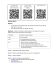### 12 Solving Percents Using Proportions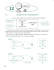### If ABC is obtuse, what are the restrictions on x? ∠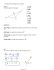### Title: Common Core State Standards Classroom Implementation for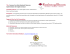### Nanocomp Pattern generators, PDF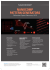### END OF COURSE GEOMETRY CORE 1 VIRGINIA STANDARDS OF LEARNING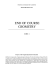### STUDY GUIDE for Unit 1 Test Geometry 1. Z?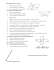### VA517571_GM Release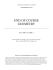### Original Green™ Diamond - Popp Dental Supply, LLC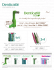### Name: ____________________________________________ 1)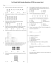### GEO6 GEOMETRY AND MEASURESUREMENT Student Pages for Packet 6: Drawings and Constructions